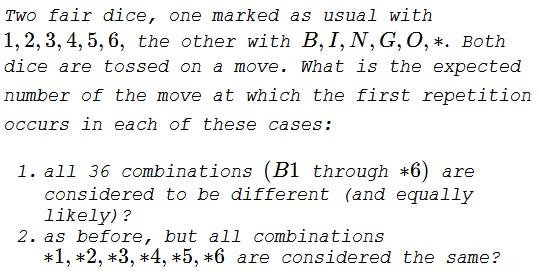# Two Dice Repetition

### Problem### Solution, Question 1

Very much like in the Birthday Problem, but with $36$ distinct equiprobable selections.

A repetition occurs on move $n,~2\le n\le 37$ for the first time if, until then, there were no repetition but then on the $n^{th}$ move there was one. The probability of this event is

\displaystyle \begin{align} p(n)&=\frac{35}{36}\cdot\frac{34}{36}\cdots\frac{36-(n-2)}{36}\cdot\frac{n-1}{36}\\ &=\frac{(n-1)36\cdot 35\cdots (38-n)}{36^n} \end{align}

The expectation for the number of moves then is

$\displaystyle E=\sum_{k=2}^{37}kp(k)\approx 8.203.$

### Solution, Question 2

Now, for $n,~2\le n\le 32,$ the first repetition can occur at move $n$ in one of two ways:

1. there was no $*$ in the first $n-1,$ or
2. there was exactly one $*$ in the first $n-1$ moves, whereas $*$ may or may not appear on move $n.$

Let $q(n)$ is the probability of the first repetitions happening on move $n.$ The, to start with,

\displaystyle\begin{align}q(2)=\frac{30}{36}\cdot\frac{1}{36}+\frac{6}{36}\cdot\frac{6}{36}=\frac{11}{216}. \end{align}

For $3\le n\le 32,$

\displaystyle\begin{align}q(n)&=\frac{30\cdot 29\cdots (32-n)}{36^{n-1}}\cdot\frac{n-1}{36}\\ &\qquad\qquad+(n-1)\cdot\frac{6}{36}\cdot\frac{30\cdot 29\cdots (33-n)}{36^{n-2}}\cdot\frac{6+(n-2)}{36}\\ &=\frac{30\cdot 29\cdots (33-n)}{36^{n}}\cdot (n-1)(5n+56). \end{align}

The expected number of moves at which the first repetition occurs is then

$\displaystyle \sum_{k=2}^{32}kq(k)\approx 6.704.$

### Acknowledgment

This is a paraphrase of problem 409 from Crux Mathematicorum (posed v 5, n 1; solved v 5, n 9, 1979). The problem and solution are by L. F. Meyers of the Ohio State.

The problem is modeled after a popular game of old copyrighted by Pop-o-Mathic.